# Analyze Data Using the XY Plot

This example shows how to analyze data plotted on an XY plot in the Simulation Data Inspector using trend lines.

The XY plot used in this example is also available in the Record block and the XY Graph block. When you use the XY plot in the Record block and the XY Graph block, you add the visualization and configure the appearance using the toolstrip. You plot data on the XY plot the same way shown in this example.

### Import Data into Simulation Data Inspector

Open the Simulation Data Inspector.

`Simulink.sdi.view`

The data for this example is stored in the MAT file `XYData.mat`. To import the data using the UI, click Import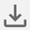.

In the Import dialog box, under Import from, select File. Then, enter `XYData.mat` in the text box and click Import.

Alternatively, import the data programmatically using the `Simulink.sdi.createRun` function.

`xyRunID = Simulink.sdi.createRun("XY signals","file","XYData.mat");`

### Plot Data on XY Plot

By default, the Simulation Data Inspector uses time plots for each subplot in the layout. To plot the data on an XY plot, you need to add the visualization to the layout.

To add an XY plot to your layout, click Layout. Then, select Edit View.From the Visualization Gallery, drag the XY icon onto the subplot.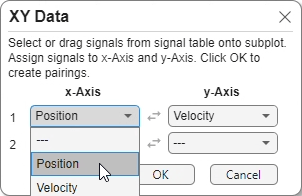Alternatively, you can modify the visualization for a subplot using the subplot menu. Pause on the subplot where you want to change the visualization, then click the three dots that appear. From the Change Visualization drop-down list, select the visualization you want to use for that subplot.To plot signals on the XY plot, select two signals in the table and drag them onto the plot. For example, select the rows for the `Heart-X` and `Heart-Y` signals, then drag them onto the plot. Hold the Shift key or the Ctrl key to select more than one signal row in the table.Specify which signal to use as x-data and which to use as y-data in the menu that appears in the lower-right of the plot. Use the first drop-down list to select the signal that provides the x-data, and use the second drop-down list to select the signal that provides the y-data. To swap the current x- and y- data selections, click the Swap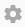button between the drop-down lists.You can modify the appearance of XY plots in the layout using the Visualization Settings. To access the visualization settings for the XY plot, click Visualization Settings.

The line and marker colors can match the color for the signal that provides the x data or the color for the signal that provides the y data. By default, the line and markers both use the y-axis signal color. Specified settings apply for all XY plots in the layout.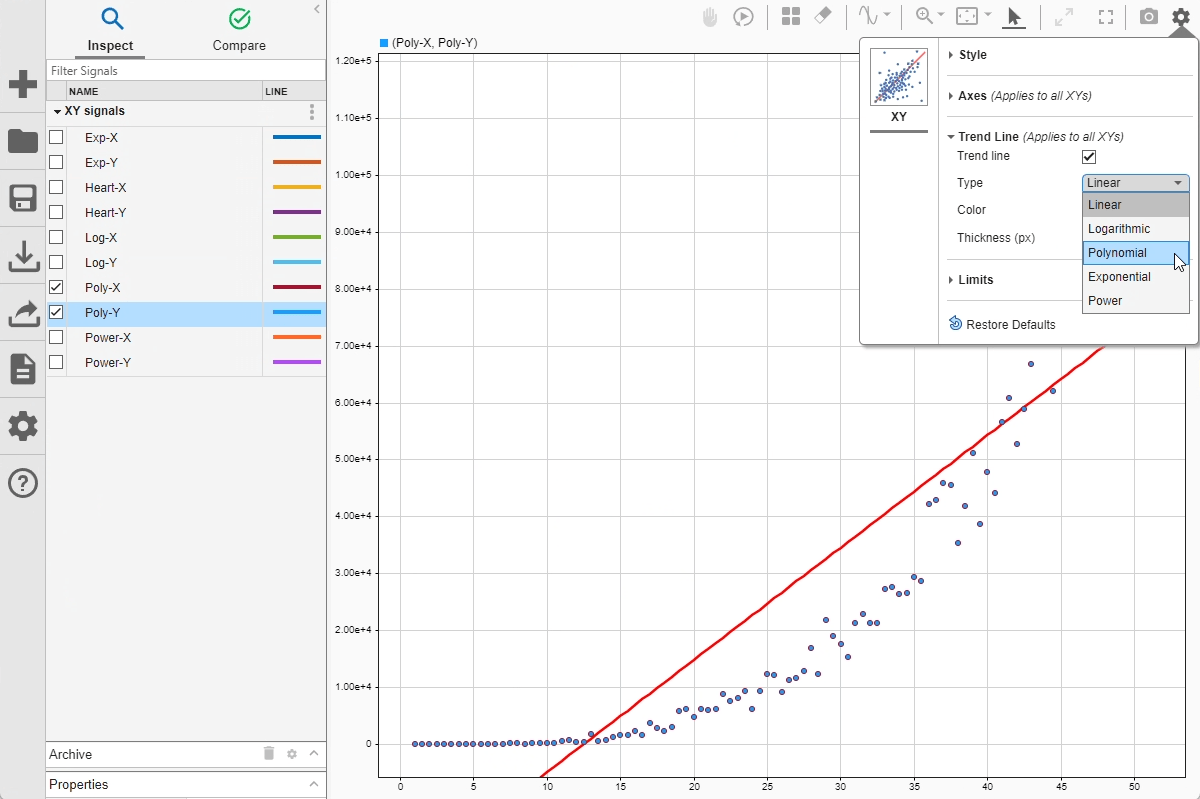### Analyze XY Data Using Trend Lines

You can add a trend line to an XY plot to analyze the relationship between the x- and y-data. The trend line is most useful when the relationship can be expressed as a function. The `Heart-X` and `Heart-Y` data plotted in the prior section is not well-suited for analysis with trend lines because the y-data is not well correlated with the x-data.

The example data includes x- and y-data well-suited for each type of trend line available. You can try plotting each pair of signals to see how each trend line helps you analyze the data. For example, plot the `Poly-X` and `Poly-Y` signals on the XY plot:

1. Select the rows for both signals in the table. Hold the Shift key or the Ctrl key to select more than one signal row.

2. Drag the selected signals onto the plot.

3. In the menu that appears in the lower-right of the plot, specify which signal to use for the x-data and which to use for the y-data. In the first row, select `Poly-X` from the first drop-down list and select `Poly-Y` from the second drop-down list.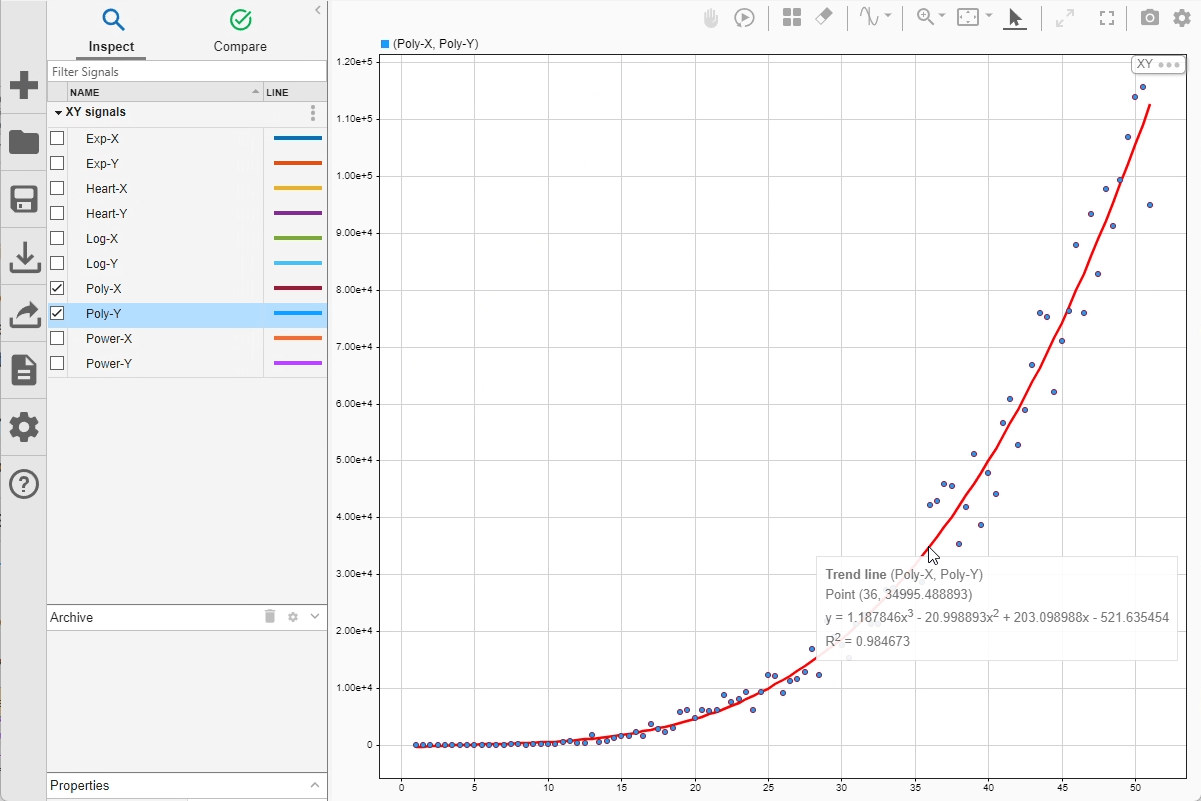4. Click OK.

To add a trend line, click Visualization Settings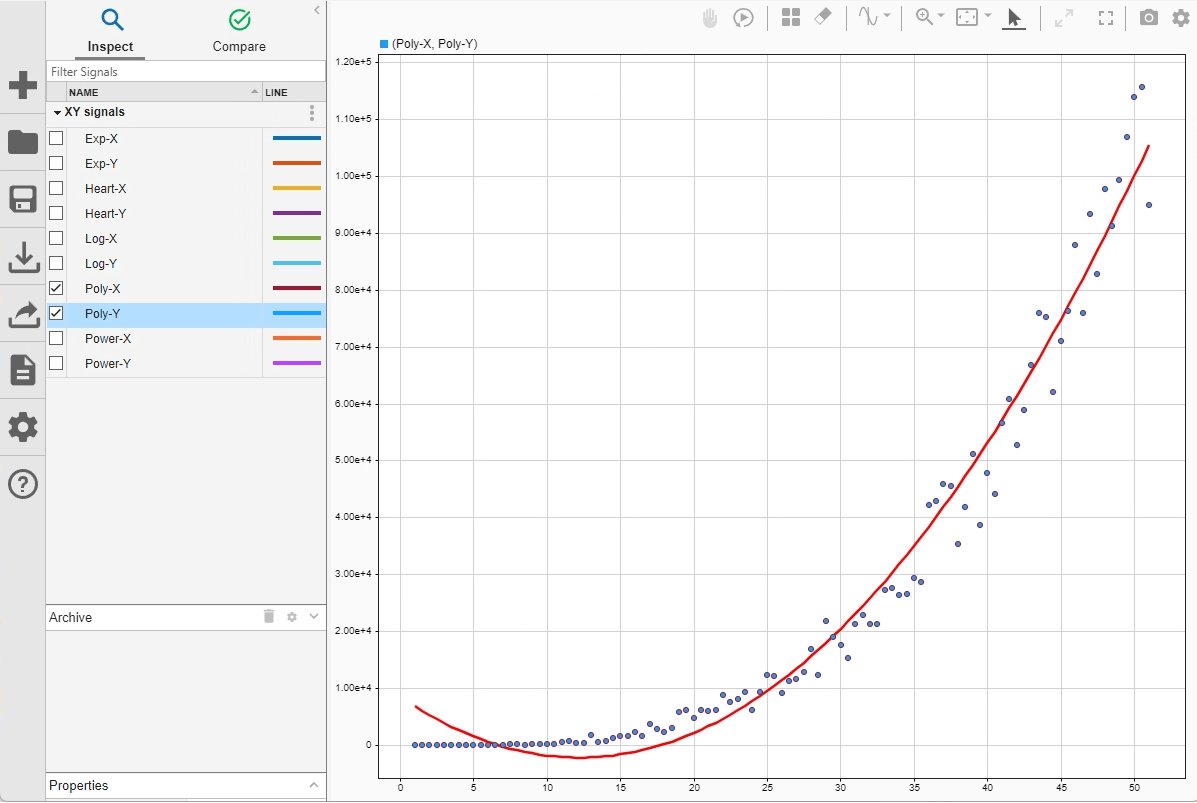and select Show trend line. From the Type list, select `Polynomial`.By default, the Simulation Data Inspector computes the polynomial trend line in the form of a third-order polynomial. To see the equation for the trend line as well as information about how it fits the data, pause on the trend line. The tooltip for the trendline shows:

• The names of the signals that provide the x- and y- data for the series.

• The coordinates for the trend line point closest to the pointer.

• The trend line equation and R-squared value.When you use a polynomial trend line, you can specify the order of the polynomial between 2 and 6, inclusive. For example, change the order of the polynomial trend line to `2` to see how it affects the fit of the trend line. Open the visualization settings for the XY plot. Then, in the text box next to the trend line type selection, enter `2`.

When you use a second-order polynomial, the trend line does not fit the start of the data as well.Try other trend line options to see how they fit the `Poly-X` and `Poly-Y` data. You can add these types of trend line to the XY plot:

• Linear: Trend line equation has the form $\mathit{y}=\mathrm{mx}+\mathit{b}$.

• Logarithmic: Trend line equation has the form $\mathit{y}=\mathit{a}\text{\hspace{0.17em}}\mathrm{ln}\left(\mathit{x}\right)+\mathit{b}$. The x-data must not contain `0` or negative values.

• Polynomial: Trend line equation has the form $\mathit{y}={\mathit{b}}_{6}{\mathit{x}}^{6}...+{\mathit{b}}_{2}{\mathit{x}}^{2}+{\mathit{b}}_{1}\mathit{x}+\mathit{a}$, where the number of terms is determined by the specified order.

• Exponential: Trend line equation has the form $\mathit{y}=\mathit{a}{\mathit{e}}^{\mathit{bx}}$. The y-data must not contain `0` or negative values.

• Power: Trend line equation has the form $\mathit{y}=\mathit{a}{\mathit{x}}^{\mathit{b}}$. The x- and y-data must not contain `0` or negative values.

Try plotting other pairs of signals in the XY plot and explore how each trend line option fits each data set.